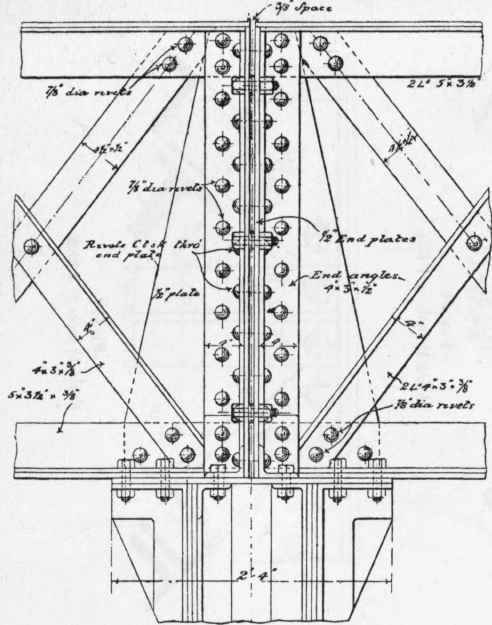As an example of this application of girderwork, details will now be given of a lattice girder of 53' span supporting a series of roof principals, the details of these principals being given in Figs. 216 and 298 to 307. Fig. 81 gives a skeleton outline of the triangulated girder the principals being carried immediately over the vertical struts, as shown, p, p, p. The girders are carried on side columns and a centre column as shown, but are not continuous, and a roof principal does not occur over the centre column. The general arrangements at side and centre columns are shown in Figs. 82, 83, while the details of connections are shown to a larger scale, for the side column in Figs. 84, 85, and 86, the latter being a section of that portion of the column to which the girder is attached, and for the centre column in Figs. 87, 88, 90. The normal section of the girder is shown in Fig. 89. The details of the apices of the triangulation, or the intersections of the web bracings with the flanges, are shown for apices 2, 4, 6, and 8 in Figs. 91, 92, 93, 94, and for apices 1, 3, 5, and 7 in Figs. 95, 96, 97, 98, showing the riveted connections. These are examples on a small scale of oblique connections, and may be studied in connection with those for roof-work shown in Chapter IV (On The Practical Design Of Columns And Struts).Fig. 81. Scale 1 inch = 16 feet.Fig. 82. Scale ⅜ inch = 1 foot.Fig. 83. Scale ⅜ inch = 11 foot.Fig. 84. Scale ¾ inch = 1 foot.Fig. 85 (Scale ¾ inch = 1 foot).Fig. 86 (Scale ¾ inch = 1 foot).Fig. 87. Scale ¾ inch = 1 foot.Fig. 88, Scale ¾ inch = 1 foot.Fig. 89. Scale ¾ inch = 1 foot.Fig. 90. Scale ¾ inch = 1 foot.Fig. 91. Scale 1½ inch = 1 foot.Fig. 92. Scale 1½ inch = 1 foot.Fig. 93. Scale 1½ inch = 1 foot.Fig. 94. Scale 1½ inch = 1 foot.Fig. 95. Scale 1½ inch 1½ = 1 foot.

In Figs. 99, 100, and 101 are given the details of the angle and flange plate joints in the girder, which occur between apices 6 and 7 in the top boom, and break joint in the bottom boom, thus dividing the girder into two lengths for convenience in transport, and keeping the lengths of angles and plates within ordinary limits.Fig. 96. Scale 1½ inch = 1 foot.Fig. 97. Scale 1½ inch = 1 foot.Fig. 98. Scale 1½ inch = 1 foot.

FIG. 99. Scale ¾ inch = 1 foot.Fig. 100. Scale ¾ inch = 1 foot.Fig. 101. Scale ¾ inch = 1 foot.

Further details of light lattice girders in connection with roof work will be found in Figs. 326, 337.